Next: Curve of Buoyancy Up: Hydrostatics Previous: Determination of Metacentric Height

# Energy of a Floating Body

The conditions governing the equilibrium and stability of a floating body can also be deduced from the principle of energy.

For the sake of simplicity, let us suppose that the water surface area is infinite, so that the immersion of the body does not generate any change in the water level. The potential energy of the body itself is, whereis the body's weight, and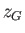the height of its center of gravity,, relative to the surface of the water. If the body displaces a volume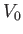of water then this effectively means that a weightof water, whose center of gravity is located at the center of buoyancy,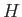, is removed, and then spread as an infinitely thin film over the surface of the water. This involves a gain of potential energy of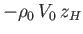, whereis the height ofrelative to the surface of the water. Vertical force balance requires that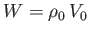. Thus, the potential energy of the system is(modulo an arbitrary additive constant), whereis the height of the center of gravity relative to the center of buoyancy.

According to the principles of statics, an equilibrium state corresponds to either a minimum or a maximum of the potential energy (Fitzpatrick 2012). However, such an equilibrium is only stable when the potential energy is minimized. Thus, it follows that a stable equilibrium configuration of a floating body is such as to minimize the height of the body's center of gravity relative to its center of buoyancy.Next: Curve of Buoyancy Up: Hydrostatics Previous: Determination of Metacentric Height
Richard Fitzpatrick 2016-03-31